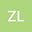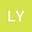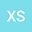Monotone iterative technique for nonlinear differential equation of fractional order
•••Li Yongjun
Lanzhou City University
Author ProfileXiaoyan Shi
Southwestern University of Finance and Economics
Author Profile## Abstract

In this paper, we mainly study the existence of solution of fractional differential equations. Firstly, the existence of the maxmum solution and minmum solution of the differential equation are proved by using the fixed point theorem and the monotone iteration method. Secondly, the existence of the solution of the original equation is proved by using the newly constructed differential equation. Finally, the application of the monotone iteration method is given through an example.